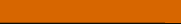A LEVEL COMPUTINGFeatures of LLLTheory

## 9. Worked example

By now you have read about many features of a low level language. Let's put together a worked example to illustrate how the instructions work together to form working code. The code below is a typical snippet of assembly language.

 .MODEL SMALL .Define the memory model to be used .STACK 2048 .Set up the stack EQU 2 Define a constant DB Define a symbol as a byte DW Define a symbol as a word DB "Hello World",0 Define a string .CODE Start of code block MOV AX,DGROUP Move an address into a word register MOV DS,AX MOV [VarB],35 Initialise variable called VarB SUB VarB,35 Subtract 35 from VarB, this will cause a zero flag in the PSW to be set JZ :mysub Jump if zero to label mysub OUT #1,3 If not zero then output data to port 1 JMP :leave Unconditionally jump to label leave IN AL,#1 Read port 1 and load into register AL MOV AL,34
##### Some notes:

The first statement .MODEL SMALL is a telling the assembler what kind of memory model to use, in this case 'small' will probably mean only a 16 bit word is needed for addressing purposes. This is faster than having to fully resolve a full 32 bit word address every time.

Then the stack is declared along with some symbolic variables.

Another declaration .CODE defines the start of the code itself.

Labels such as main, mysub and leave are used to identify specific points within the code

There are a number of MOV commands to shuffle data around

There is a SUB arithmetic command that alters the value of the variable varB

There is a conditional jump JZ (JZ :mysub) that is testing the outcome of the prior operation. This code is artificial as VarB was initialised with 35 and then 35 was subtracted, so the result will always be zero. Or this is may be an example of a typical software bug where the programmer did not intend to do that.

You can also see an unconditional JMP command (JMP :leave) to by-pass the instruction at mysub.

Challenge see if you can find out one extra fact on this topic that we haven't already told you

Click on this link: example assembly language